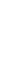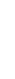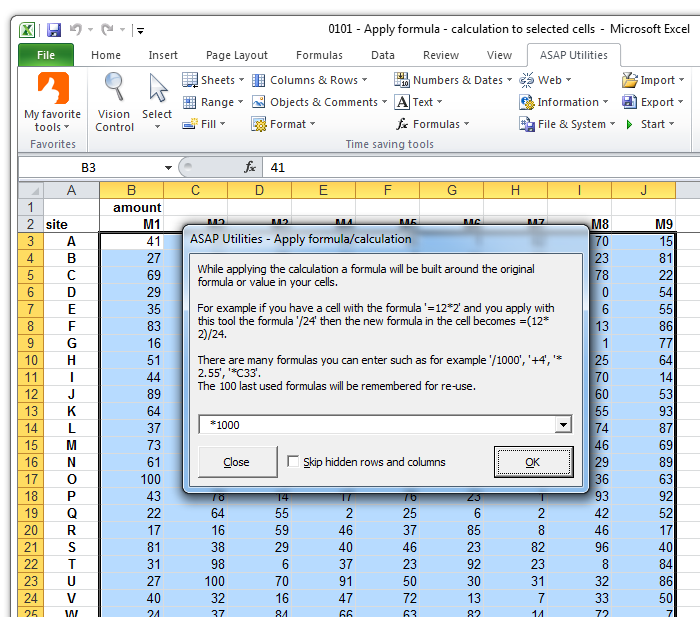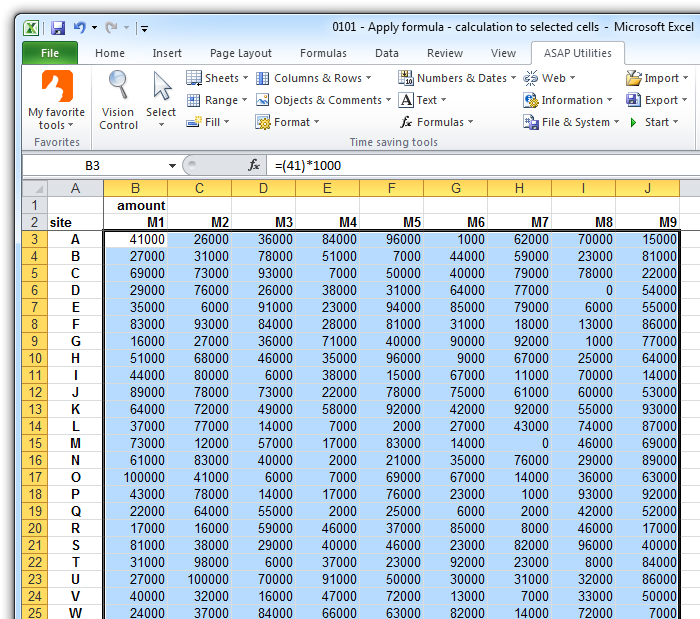# ASAP Utilities, description of our Excel toolsEnglish (us) / Nederlands / Deutsch / Español / Français / Português do Brasil / Italiano / Русский / 中文(简体) / 日本語

## Formulas » Apply formula/calculation to selected cells...

With this utility you can quickly perform a calculation on the selected range.
For example quickly multiply all cells in your selection by 10 without having to add an extra column and enter a formula to do this.

A formula will be built around the original formula or value in your cell. For example if you have a formula '=(12*2)' in a cell and you use ASAP Utilities to apply the formula /24 the result will be: '=(12*2)/24'.
The formula will be applied to all cells in your selection except for cells with errors, empty cells, cells contain text or cells that contain an array formula.
If the new formula would result in an error you will get a warning upfront and no changes will be applied.

Users of the non-English Excel versions can type in the formula in their local language. For example in the Dutch version you can use the comma as decimal separator and the local version of worksheet formulas, for example '+SOM(G20:G40)' instead of the English '+SUM(G20:G40)'.
There are many formulas you can enter such as for example '/1000', '+4', '*2.55', '*C33'.
This utility is also often used for unit conversions, such as for example converting kilometers to meters.

The last 100 used formulas are remembered so you can easily re-use them.
By default this can be started with the shortcut Ctrl+Alt+I.

## Screenshots

Example screenshot: 1 Perform a quick calculation in selected cellsExample screenshot: 2 The resultPractical 'real world' examples on our blog that show you how this tool can help you in Excel. Enjoy!

## Starting this utility

In the Excel menu choose: ASAP Utilities » Formulas » Apply formula/calculation to selected cells...